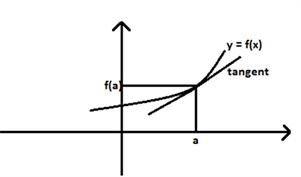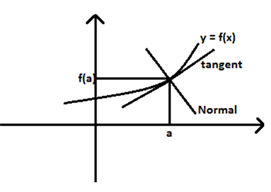# Tangent - Equation Of Tangent And Normal

The applications of derivatives are:

• determining rate of change of quantities,
• finding the equations of tangent and normal to a curve at a point,
• finding turning points on the graph of a function which in turn will help us to locate points at which largest or smallest value (locally) of a function occurs.

In this article, we will learn to use differentiation to find the equation of the tangent line and the normal line to a curve at a given point.

## Tangent Line to a Curve

Tangent is a straight line which touches the curve at a given point and it has the same slope of the curve at that point.

Consider the function y = f(x) given in the figure,First derivative of a function at a point gives its slope of the tangent at that point.

For the function f, first derivative i.e. f’at the point where x =a  is f‘(a).

f‘(a) = slope of the tangent at the point where x =a

Equation of a straight line $\frac {(y-y_1)}{(x-x_1)}$ = m , where “m” is slope of the line.

$\frac{(y – f(a))}{(x-a)}$= f’(a) is the equation of tangent of the function at x=a.

Example:

Consider the function f(x) = x3-3x2+2x+1, . Find equation of tangent of f(x)when . x = 2.

f(x) = x3-3x2+2x+1

f(2) = (2)3-3(2)2+2(2)+1

First derivative of the function f(x).f‘(x) = d/dx(x3-3x2+2x+1)

= 3x2 – 6x + 2

f‘(2)= 3 × 22 – 6 × 2 + 2 = 12 – 12 + 2 = 2

Equation of the tangent = $\frac {(y-1)}{(x-2)}$ = 2.

y – 1 = 2(x – 2)

y – 1 = 2x – 4

y = 2x – 3 is the equation of tangent.

## Normal to a curve

Normal is a line which is perpendicular to the tangent to a curve. Tangent and normal of f(x) is drawn in the figure below.Since tangent and normal are perpendicular to each other, product of slope of the tangent and slope of the normal will be equal to -1.

(y – f(a))/(x-a)} = f‘(a); is the equation of tangent of the function y = f(x) at x = a .

Then, equation of the normal will be,$\frac{(y – f(a))}{(x-a)}$ = $\frac {-1}{f'(a)}$

Example:

Consider the function,f(x) = x2 – 2x + 5 . Find the equation of tangent and equation of normal at x = 3.

f(x) = x2 – 2x + 5

f(3) = 32 – 2 × 3 + 5 = 9 – 6 + 5 = 8

First derivative, f‘(x) = d/dx(x2 – 2x + 5) = 2x – 2

f(‘x) when x = 3,f(3) = 2 × 3 – 2 = 6 – 2 = 4

Equation of the tangent is, (y-8)/(x-3) = 4

y – 8 = 4(x – 3)

y – 8 = 4x – 12

y = 4x – 4, is equation of tangent.

Slope of normal = – 1/slope of tangent = -1/4

Equation of normal is, (y-8)/(x-3) = -1/4

4(y – 8) = (3 – x)

4y – 32 = 3 – x

y = -x/4+35/4

### Points to Remember

• If a tangent line to the curve y = f (x) makes an angle θ with x-axis in the positive direction, then dy/dx = slope of the tangent tan = θ.
• If the slope of the tangent line is zero, then tan θ = 0 and so θ = 0 which means the tangent line is parallel to the x-axis. In this case, the equation of the tangent at the point (x0, y0) is given by y = y0
• If θ →π/2 , then tan θ → ∞, which means the tangent line is perpendicular to the x-axis, i.e., parallel to the y-axis. In this case, the equation of the tangent at (x0, y0) is given by x = x0

## Tangent and Normal lines Problems with Solutions

Q.1: Find the slope of the tangent to the curve y = x2+25 at x = 5
Solution: Given, y = x2+25
On differentiating the given expression, we get;
dy/dx = 2x
dy/dx = y’ = y'(5) = 2 x 5 = 10

Hence, the slope of the tangent to the curve is 10.

Q.2: Find the equation of lines having slope 2 and being tangent to the curve y + 2/(x-1)2

Solution: Slope of the tangent to the given curve at any point (x,y) is given by:

dy/dx = 2/(x-1)3

But the slope is given here as 3. Hence,

2 = 2/(x-1)3

(x-1)3 = 1

x – 1 = 1

x = 2

If x = 2, then y = -2.

Hence the equation of tangent passing through the point (2, -2), is given by;

y + 2 = 2(x-2)

y – 2x + 4 = 0